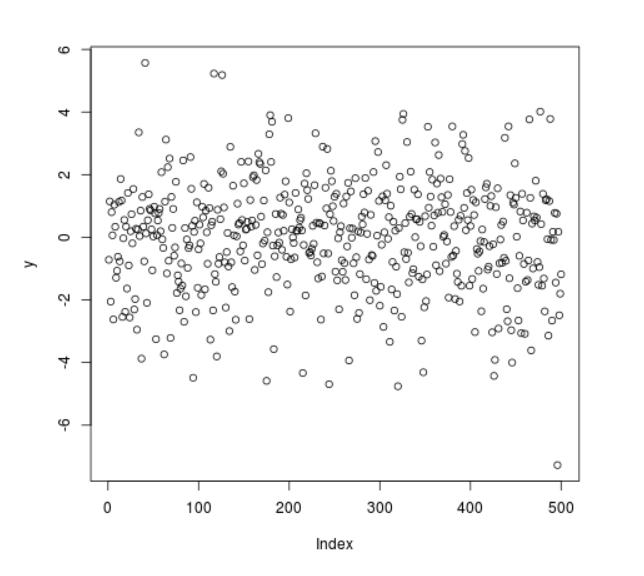Related Articles
Compute Randomly Drawn Logistic Density in R Programming – rlogis() Function
• Last Updated : 25 Jun, 2020

`rlogis()` function in R Language is used to compute random logistic density among a range of inputs.

Syntax: rlogis(N)

Parameters:
N: Sample Size

Example 1:

 `# R program to generate  ` `# random logistic density points ` ` `  `# Setting seed for ` `# random number generation ` `set``.seed(``1000``) ` ` `  `# Set sample size ` `N <``-` `20` ` `  `# Calling rlogis() Function ` `y <``-` `rlogis(N) ` `y  `

Output:

```  -0.71779506  1.14636578 -2.05114837  0.80365206  0.06563317 -2.62196720
  1.03929969  0.33730220 -1.29048105 -1.06622107 -0.61931410  1.12795649
 -0.76720400  1.86443303  1.17562774 -2.54319669 -0.02953117  0.55442681
 -2.37810163  0.33889069
```

Example 2:

 `# R program to generate  ` `# random logistic density points ` ` `  `# Setting seed for ` `# random number generation ` `set``.seed(``1000``) ` ` `  `# Set sample size ` `N <``-` `500` ` `  `# Calling rlogis() Function ` `y <``-` `rlogis(N) ` ` `  `# Plot a graph ` `plot(y) `

Output:My Personal Notes arrow_drop_up
Recommended Articles
Page :